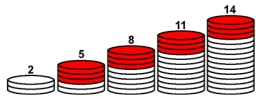# Measurements: 44341

The following roller lengths (in millimeters) were determined by laboratory measurements:
{402; 410; 412; 410; 413; 418; 405; 409; 410; 409}
Calculate arithmetic, geometric mean, mode, and median.

a =  409.8
g =  409.7796
m1 =  410
m2 =  410

### Step-by-step explanation:Did you find an error or inaccuracy? Feel free to write us. Thank you!

Tips for related online calculators
Looking for help with calculating arithmetic mean?
Looking for a statistical calculator?
Do you want to convert length units?

#### Grade of the word problem:

We encourage you to watch this tutorial video on this math problem: